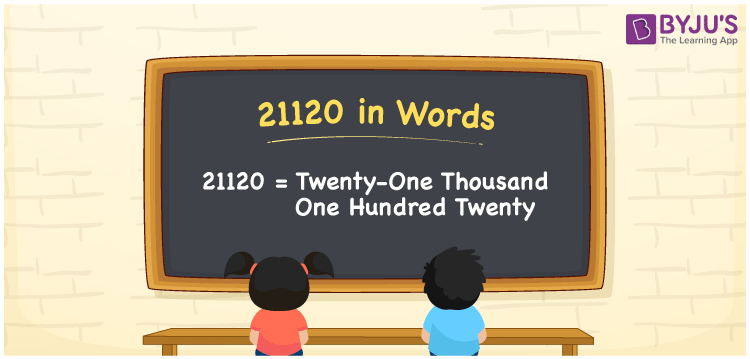21120 in words is Twenty-One Thousand One Hundred Twenty. For instance, Leena earned Rs. 21120 in a month, then you can write, “Leena earned Rupees Twenty-One Thousand One Hundred Twenty in a month”. The number 21120 is a cardinal number since it shows a specific quantity. We can convert the number 21120 in an easy manner with the help of a place value chart. Now, let us look at how to convert the number 21120 into words as well as its spelling in English words.

 21120 in Words Twenty-One Thousand One Hundred Twenty Twenty-One Thousand One Hundred Twenty in numerical form 21120

## 21120 in English Words

Generally, in Mathematics, we write the numbers in words using the English alphabet. Therefore, we can write the number 21120 in words as Twenty-One Thousand One Hundred Twenty.## How to Write 21120 in Words?

We can convert the number 21120 into words with the help of a place value chart. The place value table for the number 21120 and its expanded form is given below.

 Ten-Thousands Thousands Hundreds Tens Ones 2 1 1 2 0

Hence, we can write the expanded form as:

2 x Ten Thousand + 1 x Thousand + 1 x Hundred + 2 x Ten + 0 x One

= 2 x 10000 + 1 x 1000 + 1 x 100 + 2 x 10 + 0 x 1

= 20000 + 1000 + 100 + 20 + 0

= 20000 + 1000 + 100 + 20

= 21120

= Twenty-One Thousand One Hundred Twenty

Therefore, 21120 in words is written as Twenty-One Thousand One Hundred Twenty

Interesting way of writing 21120 in words

2 = Two

21 = Twenty-One

211 = Two Hundred and Eleven

2112 = Two Thousand One Hundred Twelve

21120 = Twenty-One Thousand One Hundred Twenty

Thus, the word form of the number 21120 is Twenty-One Thousand One Hundred Twenty

21120 is a natural number that is the successor of 21119 and the predecessor of 21121

• 21120 in words – Twenty-One Thousand One Hundred Twenty
• Is 21120 an odd number? – No
• Is 21120 an even number? – Yes
• Is 21120 a perfect square number? – No
• Is 21120 a perfect cube number? – No
• Is 21120 a prime number? – No
• Is 21120 a composite number? – Yes

## Frequently Asked Questions on 21120 in Words

Q1

### Write 21120 in words.

21120 in words is Twenty-One Thousand One Hundred Twenty.
Q2

### Simplify 20000 + 1120, and express in words.

Simplifying 20000 + 1120, we get 21120. Thus, the number 21120 in words is Twenty-One Thousand One Hundred Twenty.
Q3

### 21120 is a prime number. True or False.

False, the number 21120 is not a prime number.